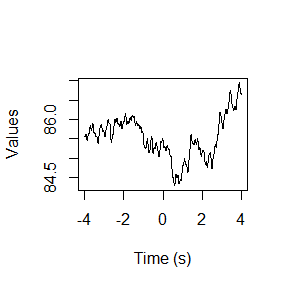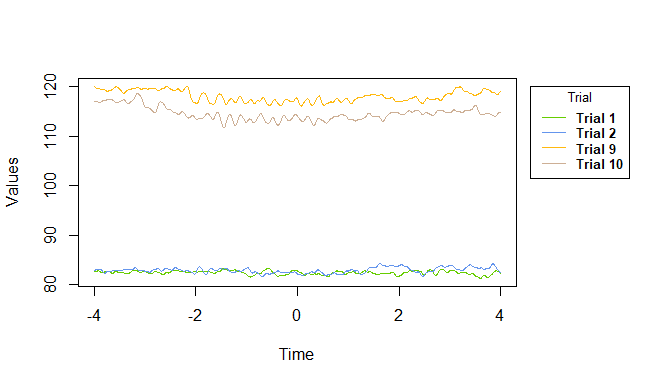Working with Waveform Data using GCalcium

2019-03-04

Calcium indicator methods such as GCaMP produce massive amounts of data; in some cases producing hundreds-of-thousands of data points for a single subject. Further, there is currently no ubiquitous way to organize or analyze this type of data. To even analyze the data, the researcher must:

• Organize the data into a format that is easy to manipulate

• Extract useful data points for visualization and analysis

• Visualize the data

The GCalcium package gets researchers to the analysis phase more quickly by:

• Providing simple, quick commands to format data for use both with, and without the GCalcium package

• Extracting data points and waveform characteristics for summarizing and comparing activity within and between trials

• Shortcuts for useful visualizations

This document explains how to use GCalcium’s functions to format, extract, and manipulate calcium indicator data.

Data: GCaMP

The data included with the GCalcium provides a sample of a time series-like dataset exported from Matlab using the TDTFilter command with a modified version of Dr. David Root’s Matlab script. This data was collected using GCaMP6.

This dataset consists of 11 rows and 814 columns. 10 trials from a pilot study were used, with calcium activity from 4 seconds before and after stimulus onset (0s).

Data formatting

In order to use the rest of the package, data must be in a data frame format, with the requirements being:

1. The first column is a measure of time

2. The following columns are recorded values from trials in ascending order that correspond to the times the values were recorded at, with 1 column per trial

Fortunately, the GCalcium package includes functions that quickly reformat the data for ease with both user manipulation and use of this package. All formatting commands output this type of data frame.

Format organized data with format_data

Currently, the only command for formatting data is format_data, which takes a matrix of a time measurement in the first column or row, and one trial per column or row in the subsequent columns/rows. A data frame with the first row “Time” and subsequent rows “Trial#” is outputted.

Note: the data frame used with the GCalcium package does not have to be labeled the same as the format_data frame. This is simply for ease of calling each trial using outside functions.

Extracting useful information for analysis

To perform analyses or explore differences in activity waveforms, one must filter and summarize the data. Knowing what wave characteristics to compare can be confusing; as many scientists do not typically work with this type of data. The following commands extract and/or summarize numbers comparisons that have been used in past research. These functions are split into 2 types: vector inputs, and matrix (format_data style) inputs.

Vector

• find_peaks looks for peaks based on inflection points and a simple filter

• inflect_points finds the peaks and valleys of waves

find_peaks

find_peaks finds peaks or valleys in waveforms by using inflection points, with filter of ‘n’ increasing/decreasing points on both sides of each inflection point. A positive numerical input for ‘n.points’ returns the indices of peaks for the input vector, while a negative value returns indices of valleys.

Let’s say we wanted to find all peaks of trial 1 that have 10 consecutive decreasing points on the left and right of each peak, and use these indices to subset the data.

inflect_points

inflect_points uses derivatives to find and label the inflection points (peaks and valleys) of a vector, along with the points between them.

The value -2 indicates a peak, 2 indicates a valley, and 0 indicates a point on the curve between -2 and 2, or vice versa.

Matrix or Data frame

• averaged_trials Averages values across trials

• avg_curve_slope gets the average slope of a curve (half wave)

• between_trial_change finds the difference in mean activity between trials

• centered_AUC finds the area under each curve

• consecutive_trial_change finds the difference in mean activity between trial n and n+1

• moving_window summarizes data within windows of time

• inflect_points_df finds provides a summary of the data between each inflection point (half wave)

• perc_baseline calculates the percent change from a user-specified baseline period

• within_trial_change finds the difference in mean activity within a trial

averaged_trials

averaged_trials averages values over each time point, across the specified trials. This is especially useful when blocking groups of trials.

Let’s say we want to plot the averaged values of trials 1-5between_trial_change

between_trial_change finds the difference in means during the same time range between sets of trials

For example: we want to see how neural activity during the trial changes after manipulating the experimental variable. The control trials are 1-5, and the experimental trials are 6-10.

consecutive_trial_change

consecutive_trial_change finds the difference in means between consecutive trials during the same time range.

For example: we want to know how much the change in activity is along trials 1-10.

inflect_points_df

inflect_points_df uses inflect_points to find the inflection points, then summarizes the data and returns a data frame with the following variables: Time, raw (input) values, inflection points, and the number of the respective curve.

In differentiating between inflect_points and inflect_points_df, notice that the purpose of this function fully corresponds to its name. The output and first input are both data frames.

perc_baseline

perc_baseline calculates the percent change from a baseline period specified by the user for all trials in the input matrix or data frame. This outputs the same object, but with values transformed to percent change from baseline. This is a good way for standardizing data within trial periods; especially when the baseline period has low standard deviations that cause inflated values in transforming into z-scores.

within_trial_change

within_trial_change finds the change in mean values between the beginning and end of the entered time ranges for a single trial.

For example: we want to know how the mean activity changes between the first two seconds before epoc (baseline) and during the trial.

Transformations and filtering

z_score

z_score transforms input values into z-scores. This also allows for a user-specified mean and standard deviation to compare distributions.

Let’s say we wanted to see how the variability of baseline and trial compare by using a mean and standard deviation from a baseline period before epoc.

Note that the return format is different from the base R ‘scale’ function, in that it does not create new attributes.

Plotting

plot_trials

plot_trials uses the base R graphics to create a quick plot of the trial waves.

For example: we want to visualize the first 2 and last 2 trialsNote: this function automatically adjusts the x- and y- axes to fit all values. It also creates a legend for the corresponding trials.